## Paging in OS-

Before you go through this article, make sure that you have gone through the previous article on Paging in OS.

We have discussed-

• Paging is a non-contiguous memory allocation technique.
• Page Table keeps track of the frames storing the pages of the process.

## Overhead in Paging-

In paging scheme, there are mainly two overheads-

### 1. Overhead of Page Tables-

• Paging requires each process to maintain a page table.
• So, there is an overhead of maintaining a page table for each process.

### 2. Overhead of Wasting Pages-

• There is an overhead of wasting last page of each process if it is not completely filled.
• On an average, half page is wasted for each process.

Thus,

Total overhead for one process

= Size of its page table + (Page size / 2)

## Optimal Page Size-

Optimal page size is the page size that minimizes the total overhead.

It is given as-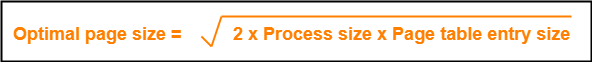Also Read- Important Formulas Of Paging

## Proof-

Total overhead due to one process

= Size of its page table + (Page size / 2)

= Number of entries x Page table entry size + (Page size / 2)

= Number of pages the process is divided x Page table entry size + (Page size / 2)

= (Process size / Page size) x Page table entry size + (Page size / 2)

Now,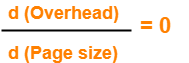Keeping process size and page table entry size as constant, differentiating overhead with respect to page size, we get-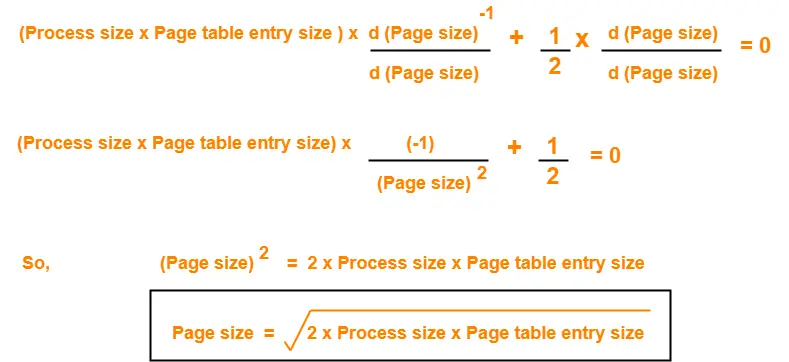## Problem-01:

In a paging scheme, virtual address space is 4 KB and page table entry size is 8 bytes. What should be the optimal page size?

## Solution-

Given-

• Virtual address space = Process size = 4 KB
• Page table entry size = 8 bytes

We know-

Optimal page size

= (2 x Process size x Page table entry size)1/2

= (2 x 4 KB x 8 bytes)1/2

= (216 bytes x bytes)1/2

= 28 bytes

= 256 bytes

Thus, Optimal page size = 256 bytes.

## Problem-02:

In a paging scheme, virtual address space is 16 MB and page table entry size is 2 bytes. What should be the optimal page size?

## Solution-

Given-

• Virtual address space = Process size = 16 MB
• Page table entry size = 2 bytes

We know-

Optimal page size

= (2 x Process size x Page table entry size)1/2

= (2 x 16 MB x 2 bytes)1/2

= (226 bytes x bytes)1/2

= 213 bytes

= 8 KB

Thus, Optimal page size = 8 KB.

## Problem-03:

In a paging scheme, virtual address space is 256 GB and page table entry size is 32 bytes. What should be the optimal page size?

## Solution-

Given-

• Virtual address space = Process size = 256 GB
• Page table entry size = 32 bytes

We know-

Optimal page size

= (2 x Process size x Page table entry size)1/2

= (2 x 256 GB x 32 bytes)1/2

= (244 bytes x bytes)1/2

= 222 bytes

= 4 MB

Thus, Optimal page size = 4 MB.

Next Article- Practice Problems On Paging in OS

Get more notes and other study material of Operating System.

Watch video lectures by visiting our YouTube channel LearnVidFun.

## Segmented Paging-

Before you go through this article, make sure that you have gone through the previous article on Segmented Paging.

We have discussed-

• Segmented Paging is a scheme that implements the combination of Segmentation and Paging.
• First, segmentation divides the process into segments.
• Then, paging divides each segment into pages.

In this article, we will discuss practice problems based on segmented paging.

## Problem-01:

A certain computer system has the segmented paging architecture for virtual memory. The memory is byte addressable. Both virtual and physical address spaces contain 216 bytes each. The virtual address space is divided into 8 non-overlapping equal size segments. The memory management unit (MMU) has a hardware segment table, each entry of which contains the physical address of the page table for the segment. Page tables are stored in the main memory and consists of 2 byte page table entries. What is the minimum page size in bytes so that the page table for a segment requires at most one page to store it?

## Solution-

Given-

• Virtual Address Space = Process size = 216 bytes
• Physical Address Space = Main Memory size = 216 bytes
• Process is divided into 8 equal size segments
• Page table entry size = 2 bytes

Let page size = n bytes.

Now, since page table has to be stored into a single page, so we must have-

Size of page table <= Page size

### Size of Each Segment-

Size of each segment

= Process size / Number of segments

= 216 bytes / 8

= 216 bytes / 23

= 213 bytes

= 8 KB

### Number of Pages Of Each Segment-

Number of pages each segment is divided

= Size of segment / Page size

= 8 KB / n bytes

= (8K / n) pages

### Size of Each Page Table-

Size of each page table

= Number of entries in page table x Page table entry size

= Number of pages the segment is divided x 2 bytes

= (8K / n) x 2 bytes

= (16K / n) bytes

### Page Size-

Substituting values in the above condition, we get-

(16K / n) bytes <= n bytes

(16K / n) <= n

n2 >= 16K

n2 >= 214

n >= 27

Thus, minimum page size possible = 27 bytes = 128 bytes.

## Problem-02:

Considering problem-01, give the division of virtual address.

## Solution-

### Number of Bits Required For Segment Number-

Number of segments the process is divided

= 8

= 23

Thus, Number of bits required to identify a particular segment in segment table = 3 bits.

### Number of Bits Required For Page Number-

Number of pages a segment is divided

= Segment size / Page size

= 8KB / 128 bytes

= 213 bytes / 27 bytes

= 26 pages

Thus, Number of bits required to identify a particular page in table = 6 bits.

### Number of Bits Required For Page Offset-

Page size

= 128 bytes

= 27 bytes

Thus, Number of bits required for page offset = 7 bits.

Thus, virtual address is divided as-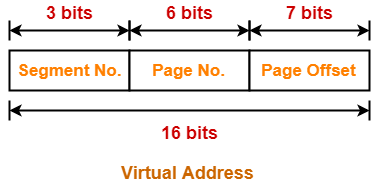## Problem-03:

A certain computer system has the segmented paging architecture for virtual memory. The memory is byte addressable. Both virtual and physical address spaces contain 216 bytes each. The virtual address space is divided into 8 non-overlapping equal size segments. The memory management unit (MMU) has a hardware segment table, each entry of which contains the physical address of the page table for the segment. Page tables are stored in the main memory and consists of 2 byte page table entries.. Assume that each page table entry contains (besides other information) 1 valid bit, 3 bits for page protection and 1 dirty bit. How many bits are available in page table entry for storing the aging information for the page? Assume that page size is 512 bytes.

## Solution-

Given-

• Virtual Address Space = Process size = 216 bytes
• Physical Address Space = Main Memory size = 216 bytes
• Process is divided into 8 equal size segments
• Page table entry size = 2 bytes = 16 bits
• Page table entry besides other information contains 1 valid bit, 3 protection bits, 1 dirty bit
• Page size = 512 bytes

### Number of Frames in Main Memory-

Number of frames in main memory

= Size of main memory / Page size

= 216 bytes / 512 bytes

= 216 bytes / 29 bytes

= 27 frames

Thus, Number of bits required for frame identification in page table entry = 7 bits

### Number Of Bits Available For Storing Aging Information-

Number of bits available for storing aging information

= Number of bits in page table entry – ( Number of bits required for frame identification + 1 valid bit + 3 protection bits + 1 dirty bit)

= 16 bits – (7 + 1 + 3 + 1) bits

= 16 bits – 12 bits

= 4 bits

Next Article- Disk Scheduling Algorithms

Get more notes and other study material of Operating System.

Watch video lectures by visiting our YouTube channel LearnVidFun.

## Segmentation and Paging-

Before you go through this article, make sure that you have gone through the previous articles on Paging and Segmentation.

We have discussed-

• Paging and Segmentation are the non-contiguous memory allocation techniques.
• Paging divides the process into equal size partitions called as pages.
• Segmentation divides the process into unequal size partitions called as segments.

## Segmented Paging-

 Segmented paging is a scheme that implements the combination of segmentation and paging.

## Working-

In segmented paging,

• Process is first divided into segments and then each segment is divided into pages.
• These pages are then stored in the frames of main memory.
• A page table exists for each segment that keeps track of the frames storing the pages of that segment.
• Each page table occupies one frame in the main memory.
• Number of entries in the page table of a segment = Number of pages that segment is divided.
• A segment table exists that keeps track of the frames storing the page tables of segments.
• Number of entries in the segment table of a process = Number of segments that process is divided.
• The base address of the segment table is stored in the segment table base register.

## Translating Logical Address into Physical Address-

• CPU always generates a logical address.
• A physical address is needed to access the main memory.

Following steps are followed to translate logical address into physical address-

## Step-01:

CPU generates a logical address consisting of three parts-

1. Segment Number
2. Page Number
3. Page Offset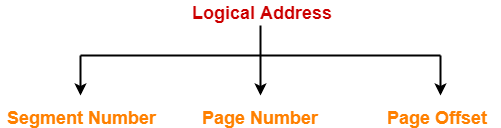• Segment Number specifies the specific segment from which CPU wants to reads the data.
• Page Number specifies the specific page of that segment from which CPU wants to read the data.
• Page Offset specifies the specific word on that page that CPU wants to read.

## Step-02:

• For the generated segment number, corresponding entry is located in the segment table.
• Segment table provides the frame number of the frame storing the page table of the referred segment.
• The frame containing the page table is located.

## Step-03:

• For the generated page number, corresponding entry is located in the page table.
• Page table provides the frame number of the frame storing the required page of the referred segment.
• The frame containing the required page is located.

## Step-04:

• The frame number combined with the page offset forms the required physical address.
• For the generated page offset, corresponding word is located in the page and read.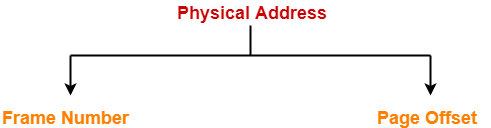## Diagram-

The following diagram illustrates the above steps of translating logical address into physical address-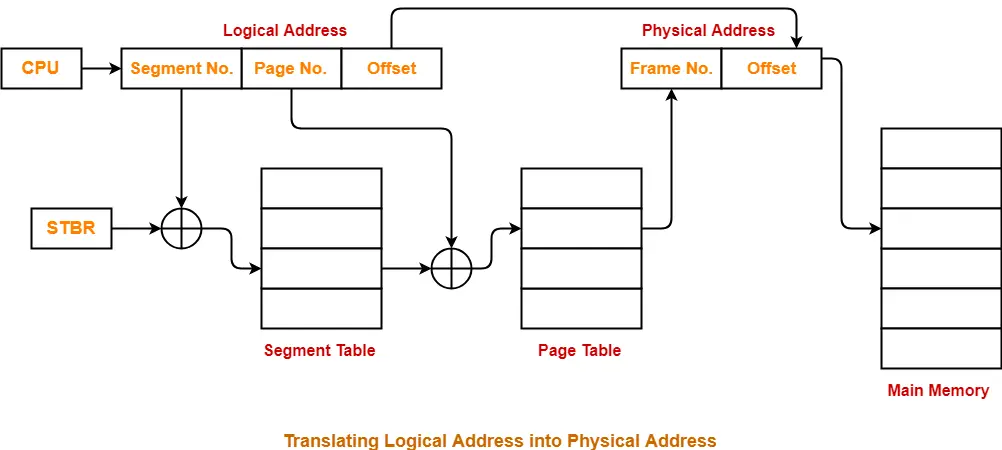The advantages of segmented paging are-

• Segment table contains only one entry corresponding to each segment.
• It reduces memory usage.
• The size of Page Table is limited by the segment size.
• It solves the problem of external fragmentation.

The disadvantages of segmented paging are-

• Segmented paging suffers from internal fragmentation.
• The complexity level is much higher as compared to paging.

Next Article- Practice Problems On Segmented Paging

Get more notes and other study material of Operating System.

Watch video lectures by visiting our YouTube channel LearnVidFun.

## Segmentation in OS-

Before you go through this article, make sure that you have gone through the previous article on Segmentation in OS.

We have discussed-

• Like Paging, Segmentation is another non-contiguous memory allocation technique.
• Segmentation divides the process into meaningful segments.
• Segment table stores the information about each segment of the process.

In this article, we will discuss a practice problem based on segmentation.

## Problem-

Consider the following segment table-

 Segment No. Base Length 0 1219 700 1 2300 14 2 90 100 3 1327 580 4 1952 96

Which of the following logical address will produce trap addressing error?

1. 0, 430
2. 1, 11
3. 2, 100
4. 3, 425
5. 4, 95

Calculate the physical address if no trap is produced.

## Solution-

In a segmentation scheme, the generated logical address consists of two parts-

1. Segment Number
2. Segment Offset

We know-

• Segment Offset must always lie in the range [0, limit-1].
• If segment offset becomes greater than or equal to the limit of segment, then trap addressing error is produced.

### Option-A: 0, 430-

Here,

• Segment Number = 0
• Segment Offset = 430

We have,

• In the segment table, limit of segment-0 is 700.
• Thus, segment offset must always lie in the range = [0, 700-1] = [0, 699]

Now,

• Since generated segment offset lies in the above range, so request generated is valid.
• Therefore, no trap will be produced.
• Physical Address = 1219 + 430 = 1649

### Option-B: 1, 11-

Here,

• Segment Number = 1
• Segment Offset = 11

We have,

• In the segment table, limit of segment-1 is 14.
• Thus, segment offset must always lie in the range = [0, 14-1] = [0, 13]

Now,

• Since generated segment offset lies in the above range, so request generated is valid.
• Therefore, no trap will be produced.
• Physical Address = 2300 + 11 = 2311

### Option-C: 2, 100-

Here,

• Segment Number = 2
• Segment Offset = 100

We have,

• In the segment table, limit of segment-2 is 100.
• Thus, segment offset must always lie in the range = [0, 100-1] = [0, 99]

Now,

• Since generated segment offset does not lie in the above range, so request generated is invalid.
• Therefore, trap will be produced.

### Option-D: 3, 425-

Here,

• Segment Number = 3
• Segment Offset = 425

We have,

• In the segment table, limit of segment-3 is 580.
• Thus, segment offset must always lie in the range = [0, 580-1] = [0, 579]

Now,

• Since generated segment offset lies in the above range, so request generated is valid.
• Therefore, no trap will be produced.
• Physical Address = 1327 + 425 = 1752

### Option-E: 4, 95-

Here,

• Segment Number = 4
• Segment Offset = 95

We have,

• In the segment table, limit of segment-4 is 96.
• Thus, segment offset must always lie in the range = [0, 96-1] = [0, 95]

Now,

• Since generated segment offset lies in the above range, so request generated is valid.
• Therefore, no trap will be produced.
• Physical Address = 1952 + 95 = 2047

Thus, Option-(C) is correct.

To watch video solution and practice other problems,

Watch this Video Lecture

Next Article- Segmented Paging in OS

Get more notes and other study material of Operating System.

Watch video lectures by visiting our YouTube channel LearnVidFun.

## Non-Contiguous Memory Allocation-

Before you go through this article, make sure that you have gone through the previous article on Non-Contiguous Memory Allocation.

We have discussed-

• Non-contiguous memory allocation is a memory allocation technique.
• It allows to store parts of a single process in a non-contiguous fashion.

## Techniques-

There are two popular techniques used for non-contiguous memory allocation-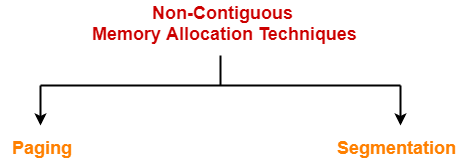## Segmentation-

• Like Paging, Segmentation is another non-contiguous memory allocation technique.
• In segmentation, process is not divided blindly into fixed size pages.
• Rather, the process is divided into modules for better visualization.

## Characteristics-

• Segmentation is a variable size partitioning scheme.
• In segmentation, secondary memory and main memory are divided into partitions of unequal size.
• The size of partitions depend on the length of modules.
• The partitions of secondary memory are called as segments.

## Example-

Consider a program is divided into 5 segments as-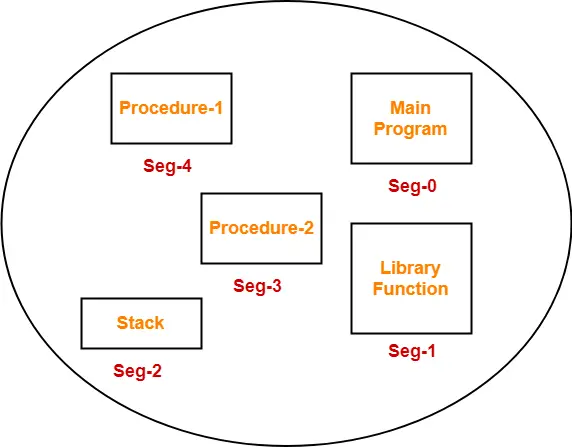## Segment Table-

• Segment table is a table that stores the information about each segment of the process.
• It has two columns.
• First column stores the size or length of the segment.
• Second column stores the base address or starting address of the segment in the main memory.
• Segment table is stored as a separate segment in the main memory.
• Segment table base register (STBR) stores the base address of the segment table.

For the above illustration, consider the segment table is-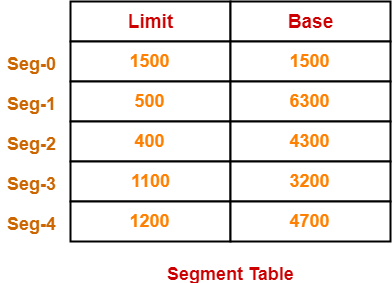Here,

• Limit indicates the length or size of the segment.
• Base indicates the base address or starting address of the segment in the main memory.

In accordance to the above segment table, the segments are stored in the main memory as-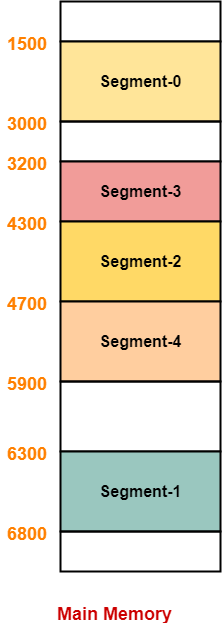## Translating Logical Address into Physical Address-

• CPU always generates a logical address.
• A physical address is needed to access the main memory.

Following steps are followed to translate logical address into physical address-

## Step-01:

CPU generates a logical address consisting of two parts-

1. Segment Number
2. Segment Offset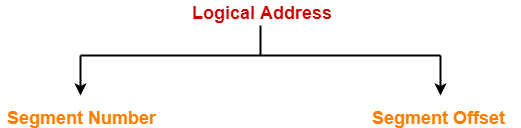• Segment Number specifies the specific segment of the process from which CPU wants to read the data.
• Segment Offset specifies the specific word in the segment that CPU wants to read.

## Step-02:

• For the generated segment number, corresponding entry is located in the segment table.
• Then, segment offset is compared with the limit (size) of the segment.

Now, two cases are possible-

### Case-01: Segment Offset >= Limit

• If segment offset is found to be greater than or equal to the limit, a trap is generated.

### Case-02: Segment Offset < Limit

• If segment offset is found to be smaller than the limit, then request is treated as a valid request.
• The segment offset must always lie in the range [0, limit-1],
• Then, segment offset is added with the base address of the segment.
• The result obtained after addition is the address of the memory location storing the required word.

## Diagram-

The following diagram illustrates the above steps of translating logical address into physical address-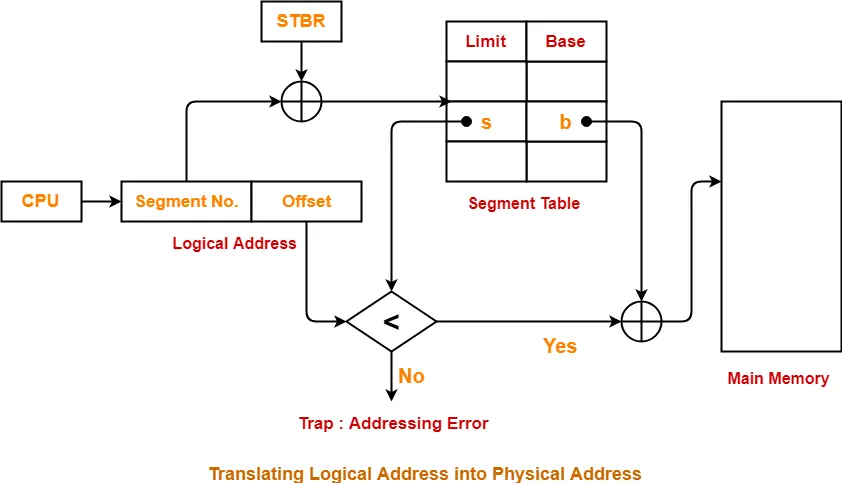The advantages of segmentation are-

• It allows to divide the program into modules which provides better visualization.
• Segment table consumes less space as compared to Page Table in paging.
• It solves the problem of internal fragmentation.

The disadvantages of segmentation are-

• There is an overhead of maintaining a segment table for each process.
• The time taken to fetch the instruction increases since now two memory accesses are required.
• Segments of unequal size are not suited for swapping.
• It suffers from external fragmentation as the free space gets broken down into smaller pieces with the processes being loaded and removed from the main memory.

To gain better understanding about Segmentation,

Watch this Video Lecture

Next Article- Practice Problems On Segmentation

Get more notes and other study material of Operating System.

Watch video lectures by visiting our YouTube channel LearnVidFun.